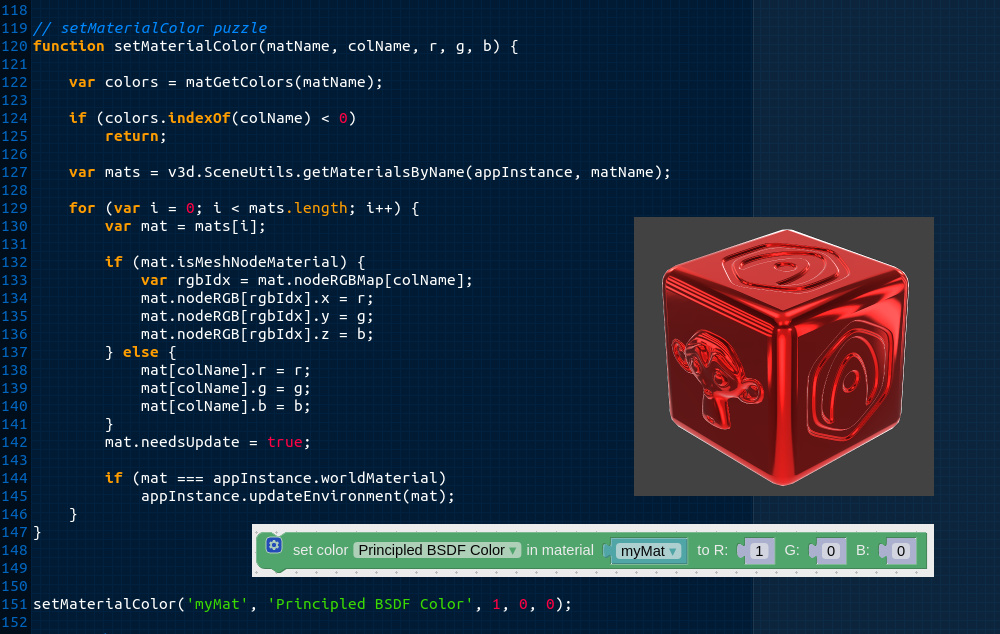# Creating Functions in JavaScript – How to Guide

JavaScript is an essential programming language for creating interactive and dynamic websites. Functions are one of the fundamental building blocks of JavaScript that allow developers to create reusable code snippets that can be called multiple times throughout a program. In this article, we will explore the basics of creating functions in JavaScript and demonstrate how they can be used to improve code efficiency and organization.

## Defining Functions in JavaScript

In JavaScript, functions are defined using the `function` keyword followed by the name of the function, a list of parameters in parentheses, and a set of curly braces containing the function’s code. For example:

``````function greet(name) {
console.log("Hello, " + name + "!");
}
``````

This function takes one parameter `name` and logs a greeting message to the console. To call this function, we simply need to use the function name followed by the parameter value(s) in parentheses. For example:

``````greet("John");
``````

This would output `Hello, John!` to the console.

## Function Parameters and Return Values

Functions can take zero or more parameters, which are values that are passed to the function when it is called. These parameters can be used within the function to perform calculations, manipulate data, or execute other code.

Functions can also return values using the `return` keyword. For example:

``````function addNumbers(a, b) {
return a + b;
}

console.log(sum); // outputs 5
``````

This function takes two parameters `a` and `b` and returns their sum using the `return` keyword. We can then assign the return value of the function to a variable and use it later in our code.

## Function Scope

In JavaScript, variables defined within a function are only accessible within that function’s scope. This means that variables defined outside of a function can be accessed by any function within the same scope, but variables defined inside a function cannot be accessed outside of that function. For example:

``````let globalVar = "I'm global!";

function myFunction() {
let localVar = "I'm local!";
console.log(globalVar); // outputs "I'm global!"
console.log(localVar); // outputs "I'm local!"
}

console.log(globalVar); // outputs "I'm global!"
console.log(localVar); // throws a ReferenceError
``````

This code defines a global variable `globalVar` that can be accessed by any function in the same scope, including `myFunction()`. However, the variable `localVar` is defined inside `myFunction()` and cannot be accessed outside of it.

## Function Expressions and Arrow Functions

In addition to the traditional `function` syntax, JavaScript also supports function expressions and arrow functions. Function expressions allow us to define functions as variables and pass them as arguments to other functions. Arrow functions are a shorthand syntax for defining functions that use the `=>` operator. For example:

``````// Function expression
const multiply = function(a, b) {
return a * b;
};

// Arrow function
const divide = (a, b) => {
return a / b;
};
``````

These two functions are equivalent to the `function` syntax we saw earlier but use alternative syntaxes.

Conclusion

Functions are a crucial aspect of JavaScript programming and allow developers to write efficient, reusable code. By understanding the basics of function syntax, parameters and return values, scope, and function expressions, you can write more complex programs with ease. With this knowledge, you can create high-quality JavaScript code that ranks highly in search results and provides a great user experience.

Scroll to Top